Thank you for visiting nature.com. You are using a browser version with limited support for CSS. To obtain the best experience, we recommend you use a more up to date browser (or turn off compatibility mode in Internet Explorer). In the meantime, to ensure continued support, we are displaying the site without styles and JavaScript.

# Prediction of variable translation rate effects on cotranslational protein folding

## Abstract

The concomitant folding of a protein with its synthesis on the ribosome is influenced by a number of different timescales including the translation rate. Here we present a kinetic formalism to describe cotranslational folding and predict the effects of variable translation rates on this process. Our approach, which utilizes equilibrium data from arrested ribosome nascent chain complexes, provides domain folding probabilities in quantitative agreement with molecular simulations of folding at different translation rates. We show that the effects of single codon mutations in messenger RNA that alter the translation rate can lead to a dramatic increase in the extent of folding under specific conditions. The kinetic formalism that we discuss can describe the cotranslational folding process occurring on a single ribosome molecule as well as for a collection of stochastically translating ribosomes.

## Introduction

Ribosome-bound nascent protein chains are particularly vulnerable to misfolding and interacting in aberrant manners with other cellular components1. To avoid these potentially dangerous possibilities and facilitate the folding process2, a variety of quality control mechanisms are associated with translating ribosomes, including those involving molecular chaperones and other ancillary factors1. An additional level of control is provided by the opportunity for proteins to fold during synthesis3,4,5,6, thus potentially enhancing folding yields7 and avoiding misfolded or intermediate species8,9. Given its importance, it is not surprising that the cotranslational folding process can be regulated by the modulation of the rates at which successive amino acids are covalently attached to the nascent chain during synthesis. Thus, for example, reduced folding yields have been observed when slow-translating messenger RNA codons are mutated to fast-translating codons10. Even single synonymous mutations have been reported to decrease the total enzymatic activity of specific types of proteins, presumably because of cotranslational misfolding11, leading to disease12. Furthermore, slow-translating codons have been observed to appear more frequently at domain boundaries10, which can result in increased folding yields. All these results indicate that the interplay of the timescales of domain folding and amino-acid addition to the nascent chain is crucial in determining the extent of cotranslational folding (Fig. 1a).Figure 1: Kinetic scheme for predicting variable translation rate effects on cotranslational folding.

Approaches based on the kinetic modelling of the molecular processes involved in translation have provided profound insights into the diverse functions of the ribosome. For example, the ability of the ribosome to discriminate between cognate and near-cognate transfer RNA has been explained using kinetic equations13,14. Here we extend this strategy to the prediction of the extent of nascent chain folding during continuous translation. This approach is based on the use of data on folding kinetics from arrested ribosome nascent chain (RNC) complexes and the time required to add individual residues to the nascent chain, quantities that can be measured using fluorescence or single molecule methods15,16. Making predictions based on arrested (that is, equilibrium) RNC data is convenient, because it is experimentally easier to probe such systems as compared with RNCs undergoing continuous, non-equilibrium translation17. Our approach is applicable to both single molecule and bulk cotranslational folding occurring during continuous protein synthesis.

## Results

### The extent of cotranslational folding on a single ribosome

To develop our approach, we first note that, in many instances, the folding of protein domains consisting of less than about 100 residues often occurs in bulk solution without significantly populating any intermediate state, and hence can be described phenomenologically by a two-state model18 (Fig. 1b). In this scheme, a protein can interconvert between folded (F) and denatured (D) states. In what follows, we will consider this model in the context of a translating protein, allowing us to predict the extent of cotranslational folding at different rates of translation.

Translation introduces the additional timescale, τA, of amino-acid addition to the two-state kinetic scheme (Fig. 1c). Because the chemical environment surrounding a protein domain changes, as it is synthesized, the timescales of its folding (τF,i) and unfolding (τD,i) are a function of the nascent chain length i, that is, the number of residues comprising the nascent chain at a particular point during its synthesis4. The time available for the domain to interconvert between folded and denatured states at length i is equal to τA,i+1, corresponding to the time it takes to attach the amino-acid i+1 to the nascent chain. τA,i+1 has been shown to be influenced by a number of factors including the identity of the mRNA codon19, the intracellular concentration of cognate and near-cognate amino-acyl-tRNAs20, and the presence of secondary structure within the substrate mRNA21. For an apparent two-state folding protein, larger τA,i+1 values will increase the probability PF(i,t) that the domain will fold (that is, achieve its native structure) by affording the domain more time to do so at a nascent chain length i and time t after initiation of synthesis (Fig. 1d).

To derive an equation relating these three timescales (τA,i+1,τF,i,τD,i) we first consider the behaviour of a single ribosome translocating along an mRNA molecule, and the time dependence of its nascent chain length. At a given nascent chain length i, the ribosome will dwell at codon i+1 waiting for this codon's cognate tRNA to be selected from the cytosol of the cell. This selection process involves a number of steps and a range of associated molecules such as elongation factor thermo unstable (EF-Tu). As we are concerned specifically with the nascent chain's length dependence as a function of time, we do not need to consider explicitly the details of these other chemical steps, for the reasons that follow. The time it takes to select the cognate tRNA and accommodate it into the A-site of the ribosome structure is stochastic in nature, but, on average, it is estimated in Escherichia coli to range from tens to hundreds of milliseconds depending on the identity of the tRNA molecule20. Once the A-site and P-site tRNAs are aligned, and receive sufficient thermal energy to pass over the transition state barrier, the chemical step of peptide bond formation, which changes the nascent chain length from i to i+1, takes on the order of picoseconds to nanoseconds22 and is known as the transition path time23,24. This six orders-of-magnitude separation in the transition path time and τA timescales (picoseconds versus milliseconds) means that, for an individual ribosome molecule, the transition from nascent chain length i to i+1 appears instantaneous relative to the time the ribosome spends at either of these chain lengths.

As a consequence, the probability, P(i), that this single ribosome molecule will contain a nascent chain of length i at time t is equal to the boxcar function probability distribution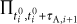which equals 1 in the time interval [ti0, ti0+τA,i+1) and is zero otherwise (Fig. 2a). ti0 is the time at which the ith amino acid is added to the nascent chain after initiation of translation. The change in P(i) with respect to time isFigure 2: Aspects of translation that are relevant in the derivation of the kinetic equation discussed in this work.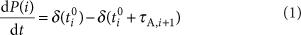where δ(t) is the Dirac delta function centred at time t after initiation of translation (Fig. 2a).

Next, we note that the experimentally observed timescale of the folding and unfolding process of a protein domain in free solution is typically on the order of milliseconds or more25, and may be much longer near the ribosome surface26. Therefore, the picosecond-to-nanosecond transition-path time of peptide bond formation will also appear as instantaneous relative to the milliseconds or more folding/unfolding timescale. As a consequence, the probability that the nascent chain is in the folded state is equal immediately before (denoted PF (i,t=ti0+τA,i+1)) and immediately after (denoted PF (i+1, t=ti+10)) the addition of the i+1 amino acid (Fig. 1d); that is, the starting point of folding at length i+1 is equal to the ending point at length i. Thus, during continuous translation, the extent of folding at a given nascent chain length is a function of the extent of folding at shorter lengths, and, hence, cotranslational folding depends recursively on what has happened at earlier times during the synthesis of the protein (Figs. 2b,c). As translation is a non-equilibrium process, memory effects can become prevalent, and so it is not surprising that the extent of cotranslational domain folding depends on the states populated at earlier times during synthesis (Fig. 2c).

The specific behaviour of a single ribosome translocating along an mRNA containing N codons is therefore characterized by the series of dwell times at each codon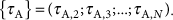If we have many independent measurements of domain folding on ribosomes that exhibit the same series of dwell times, then we can treat the domain folding probability as continuous and write down the differential equation defining the domain folding probability with respect to time as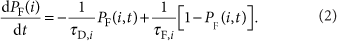and its solution is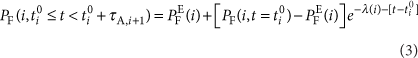We substitute equation (3) into the recursive equations shown in Fig. 2c and rearrange them to find that PF at arbitrary nascent chain length i and time t isIn equations (3) and (4),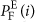is the equilibrium probability of folding and equals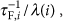with λ(i) being the rate of interconversion of the folded and denatured states that equals [τF,i]−1+[τD,i]−1. This is in contrast to the out-of-equilibrium quantityin equation (3), which is the folding probability immediately after adding the ith residue to the nascent chain. τF,i and τD,i are the average times of folding and unfolding at nascent chain length i on an arrested RNC. The placement of the first residue (i=1) in the P-site of the ribosome, corresponding to fmet-tRNA in prokaryotes27, is designated as time point zero, t10=0 s, and the time at which the ith residue is added is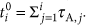### Accurate prediction of individual codon translation rate effects

Equation (4) is a function solely of τF,i, τD,i,τA,i and is a closed form solution to differential equations (1) and (2); therefore it provides an exact solution to the kinetic model shown in Fig. 1c. This equation expresses the probability that a domain is folded at each codon during continuous translation in terms of the equilibrium quantitiesand λ(i) that can be measured on arrested ribosomes, and the translation time of each codon (τA,i), which can be measured by FRET and laser optical tweezer methods15,16. To date, however, few such measurements at different nascent chain lengths have been reported. Therefore, to test equation (4) rigorously, we generated an independent data set representing the probability of domain folding at various translation rates using coarse-grained molecular simulations (Supplementary Methods) of the synthesis of protein G on the ribosome from Thermus thermophilus (Fig. 1a).

Protein G is a single domain protein whose folded architecture consists of an α-helix located adjacent to a four-stranded β-sheet platform28. The coarse-grained model that we use has been shown previously to be consistent with a range of experimental data from arrested RNC complexes4,29. As in analogous experiments17, we attached an unstructured linker to the carboxy terminus of protein G (Fig. 3a) to allow folding and unfolding of this domain to occur near the exit tunnel vestibule, where nascent chain tertiary interactions are sterically permitted29,30.Figure 3: Probability of cotranslational folding as a function of the translation rate.

We first calculated the equilibrium folding and unfolding kinetics (that is, τF,i and τD,i) of protein G on arrested RNCs containing nascent chain lengths ranging from 81 to 92 AA (Fig. 3b). These timescales can be seen to vary with the nascent chain length, a result attributable to the change in chemical environment around the domain that arises in the simulations from electrostatic and excluded volume interactions between the nascent chain and the ribosome surface. We then simulated the continuous translation of protein G by covalently attaching new glycine residues to the nascent chain's C terminus at the biologically relevant20 constant time intervals of 60, 10, 5, 2.5, and 1.3 ms, starting from a nascent chain length of 71 AA; at this length, protein G is unfolded on the ribosome as the C-terminal portion of the domain is in the exit tunnel4. To obtain statistically significant results, we carried out between 32 and 384 independent protein synthesis simulations at each translation rate.

The effects of translation rate on the extent of protein G folding at each nascent chain length are shown in Fig. 3c, and the corresponding root-mean-squared deviations of the protein G domain from its X-ray structure are shown in Fig. 4. We observe, consistent with previous conjectures31, that the greater the translation rate the smaller the probability that the domain is folded at a given nascent chain length. Furthermore, at synthesis times close to the average value in E. coli, that is, τA=50 ms, we find that continuous translation and arrested RNCs result in the same extent of folding as a function of nascent chain length (Fig. 3c). This result occurs because the folding of protein G, during continuous translation at τA=60 ms, occurs under quasi-equilibrium conditions, where the folding reaction is under thermodynamic control, whereas at τA=1.3 ms cotranslational folding occurs under non-equilibrium conditions, where folding is under kinetic control4. It is important to emphasize that domains that fold on timescales of greater than 50 ms are more likely to be under kinetic control at synthesis timescales of τA≤50 ms (τF=2 ms for protein G in free solution25), and hence show a deviation between the non-equilibrium and equilibrium folding curves PF(i, t) and. In a database of single domain folding timescales25 under physiologically relevant conditions, a quarter of them have τF≥50 ms. Thus, at average E. coli synthesis rates during exponential growth32, cotranslational folding of 25% or more of domains in multidomain proteins may be under kinetic control.

Importantly for the purpose of this study, the data in Fig. 3c provide a means to test the accuracy of equation (4). Inserting the arrested RNC folding kinetics from Fig. 3b into equation (4) and setting τA to the corresponding value used in the simulations, we find this kinetic formalism accurately and rapidly predicts the extent of cotranslational folding as a function of the translation rate (Fig. 3c). Thus, our approach captures the interplay of translation rate and folding and denaturation timescales and its consequence for the extent of cotranslational folding.

To test the sensitivity of equation (4) to single codon mutations that locally alter the translation rate along an mRNA molecule, we simulated cotranslational folding of protein G when a single 'fast'-translating codon (τA,87=1.3 ms) was placed at codon 87 in the context of a 'slow'-translating mRNA sequence (τA=10 ms). Conversely, we also simulated a system in which a single 'slow'-translating codon (τA,90=10 ms) was placed at codon 90 in the context of a 'fast'-translating mRNA sequence (τA=1.3 ms). We find that equation (4) accurately predicts the change in the extent of domain folding that results from the change in single synonymous codon mutations (Fig. 5a). This is a crucial demonstration of the utility of this formalism as synonymous mutations have been shown to alter folding yields dramatically19. These results also demonstrate that the predictions from this kinetic formalism are accurate and sensitive to the effect of variable translation rates at the level of single codons.Figure 5: The effects of synonymous codon mutations on cotranslational folding are accurately predicted by equation (4).

While the folding probabilities are shown as a function of nascent chain length in Figs 3c and 5a, equation (4) can also accurately predict these folding curves as a function of the time after the initiation of translation (Fig. 5b).

### Application to a collection of translating ribosomes

In the preceding treatment, we considered a single ribosome molecule translocating along an mRNA molecule. Equation (4) therefore represents the average domain folding probability of a nascent chain on a ribosome that translocates with a specific series of dwell times {τA}. As translocation of a ribosome along mRNA is stochastic, with a distribution of amino-acid addition times at a codon i, experiments on different ribosomes can yield different series of dwell times while they translate the same mRNA sequence.

How can we combine the exact result of equation (4), which utilizes a specific series of dwell times, with the stochastic nature of an ensemble of ribosomes, each with their own respective series of dwell times? If the probability density function Pi(τA) of amino-acid addition times at codon i is known a priori, then for a specific series of N dwell times, labelled as set k ({τA}k), we can calculate the probability pk of that series occurring by random chance as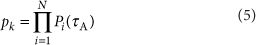Therefore, by inserting the same series of dwell times in both equations (4) and (5) , and multiplying the result as pkPF(i=N, tN0+τA,i+1), we obtain the contribution of the PF(i) folding curve of a single translating ribosome (for example, Fig. 3c) to the folding curve that would result from averaging over a large number of independent, stochastically translating ribosomes.

This result is useful for three reasons. First, equation (5) allows for the calculation of the probability of obtaining a particular single molecule trace (defined by the set of dwell times) in an experiment. Second, it allows for the numerical simulation of an arbitrarily large number of independent, stochastically translating ribosomes and each of their corresponding cotranslational folding probability curves. And finally, with sufficient such simulations, the distribution of folding probability curves and their average can be calculated for an ensemble of stochastically translating ribosomes. Importantly, this approach can be applied to arbitrary Pi(τA) distributions, thus providing it significant versatility.

To illustrate these points, consider an amino-acid addition time distribution Pi(τA) that is exponentially distributed and is therefore equal to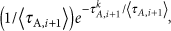where ‹τA,i+1› is the average time required for amino-acid addition to a nascent chain of length i. Values of this time have already been estimated for all 48 codons in E. coli20. τkA,i+1 is the time it takes to add the i+1 residue to the nascent chain in the kth experiment in which a single ribosome translocating along mRNA is monitored. If N=91, as in the protein G construct discussed above, and ‹τA,i+1› is taken as 60 ms for all codons, then the probability of observing a single ribosome translate a protein in which it dwells at each codon for 20 ms is effectively zero (about 10−171). To simulate the individual folding curves of 1,000 ribosomes stochastically translating this protein G construct; however, we can randomly sample τA values from the exponentially distributed Pi+1(τA) for each codon (see Methods) and construct 1,000 unique dwell time sets [{τA}k]. For each τA set, we can use equation (4) to calculate the resulting folding curve. Fig. 4 shows these 1,000 folding curves as a function of time (Fig. 6a) and nascent chain length (Fig. 6b). These results show that the kinetic model that we described can be utilized to predict how amino-acid timescales and their underlying distribution affect the extent of cotranslational folding of a protein domain at the resolution of an individual ribosome molecule, or for a large collection of ribosomes.

### Exact solution for a collection of ribosomes

When Pi+1(τA) is exponentially distributed, it is possible to derive an exact expression relating the average cotranslational folding curve from a collection of stochastically translating ribosomes as a function of nascent chain length (equation (6), Attila Szabo, personal communication). That is, the blue line in Fig. 6b can be predicted without having to resort to the numerical simulations discussed in the previous section, although, by doing so, the information on the underlying distribution of folding curves is lost.

To derive the ensemble averaged folding curve as a function of nascent chain length, denoted ‹PF(i)›, a probabilistic approach can be utilized to analyse the elementary reaction steps in Fig. 1c (see Methods). Under these conditions,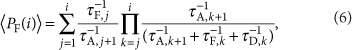where the superscript of '−1' indicates the reciprocal of these timescales. To test the accuracy of equation (6), we used it to calculate ‹PF(i)› for protein G and compared it with results from the numerical simulations described in the previous section. We find excellent agreement between this exact result and the numerical simulations (Fig. 6b). Thus, equation (6) can predict the effect of per codon translation rates on the average cotranslational folding curve that arises from bulk experiments.

## Discussion

We have presented two equations (equations (4) and (6)) that predict the extent of cotranslational domain folding based on per codon translation timescales, and the timescales of domain folding and denaturation on arrested RNC complexes at equilibrium (Fig. 3b). We have derived an exact expression for the domain folding probability in the case of a single translating ribosome (equation (4)), and shown how this expression can be utilized to predict the behaviour of a large number of stochastically translating ribosomes. Finally, an exact expression for the cotranslational folding curve was derived for ribosomes translating with exponential dwell times at each codon (equation (6)).

The utility of each of these equations depends on the questions that one is interested in addressing and the type of experiment (bulk versus single molecule) that is being carried out. In analysing and predicting cotranslational folding behaviour on individual ribosomes, equation (4) is perhaps the most relevant. The application, via numerical methods, of equation (4) to a collection of stochastically translating ribosomes is of direct consequence to both single molecule and bulk experiments as this approach offers the ability to calculate the individual ribosome folding curves as well as the ensemble average. This numerical approach can handle arbitrary distributions of amino-acid addition timescales and is thus not limited to the exponential dwell time distributions. Bulk experiments, where the average cotranslational folding curve as a function of nascent chain length may be measured from a collection of ribosomes, can be predicted using equation (6). Thus, these equations are applicable under a wide range of conditions.

Laser optical tweezers have recently26 been used to measure the folding rate under tension of T4-lysozyme arrested on the ribosome at two different linker lengths. While the unfolding rate at zero force was not estimated at either length, these experiments clearly demonstrate that it is possible to measure τF,i and τD,i experimentally, which are key inputs in our approach. We expect that, as more studies measuring these rates are carried out on this and other proteins, such data, when combined with our approach, will be useful in predicting what happens during continuous translation.

A number of additional translation-associated processes were not explicitly considered in the reaction scheme (Fig. 1c). For example, the competitive (and reversible) binding of near- and non-cognate tRNAs for a codon can slow down the rate of amino-acid addition by cognate tRNA molecules20. Furthermore, chaperones such as trigger factor directly interact with nascent chains during their synthesis, and can slow the rate of cotranslational folding of at least some proteins33. These processes do not diminish the utility of our approach, because each of these additional processes can effectively be accounted for by incorporating them into the timescales of amino-acid addition (in the case of competitive binding) and into the rates of folding and unfolding (in the case of trigger factor). The mathematical dependence of τA on near-cognate and non-cognate tRNA concentrations and their competitive binding rates has been worked out previously20. Thus, combining that model with equation (4) provides a means to model the effect of competitive tRNA binding on cotranslational folding. Similarly, when quantitative experimental measurements become available for the effect of trigger factor on the rates of domain folding and unfolding, they can be incorporated implicitly into effective timescales in these equations.

The kinetic models that we have described here are based on domains that fold cotranslationally in a two-state manner. This property applies to a variety of small proteins (typically ≤100 residues) and enables an analytical solution to be obtained for the kinetics of cotranslational folding. For protein domains larger than those examined here, which may populate intermediate states on the ribosome, the kinetic scheme in Fig. 1c can be modified to account for such additional states as they are experimentally identified. Although the additional complexity of such kinetic schemes may make it difficult to find an analytical solution, they could always be solved by numerical methods.

The approach we have proposed here has many potential applications in the areas of in vivo protein folding, biotechnology, and synthetic biology. For example, when coupled with models of translation rates that account for codon usage and tRNA concentrations34, this formalism provides a means to predict cotranslational folding behaviour of entire proteomes under varying cellular conditions and aid in the design and of synthetic transcriptomes that optimize the extent of cotranslational folding. Equations (4) and (6) also provide a way for experimentalists to map directly the results from more easily studied arrested RNCs to the realistic situation of continuous translation. Thus, such kinetic modelling of the ribosome, when combined with a variety of different experimental data, provides new research avenues and the potential for novel insights in a number of different areas.

## Methods

### An exact solution for a collection of translating ribosomes

An equation can be derived relating the domain folding probability immediately before the addition of the next amino acid to the nascent chain for a ribosome that dwells with an exponential waiting time distribution at each codon (equation (6), Attila Szabo, personal communication). This probability, denoted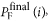is equal to the probability of taking the pathway in Fig. 1c Fi→Fi+1 and can be calculated as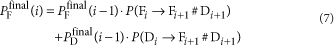where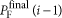and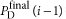are, respectively, the probabilities that, when the nascent chain changes from length i−1 to i, the domain was either in the folded or denatured state.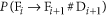is the probability, that, beginning in the folded state at length i, the RNC complex will reach the folded state at length i+1 before reaching the denatured state at length i+1. Likewise,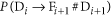is the probability that, beginning in the denatured state at length i, the system will reach the folded state at length i+1 before reaching the denatured state at length i+1. Because there are only two states in our reaction scheme (Fig. 1c), we have that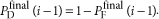Substituting this into equation (7), we have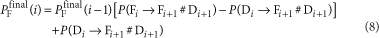and we see that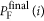is a recursive relationship.

Using the probabilistic method35 for calculating pathway probabilities in reaction schemes,andcan be easily shown to equal, respectively,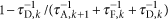and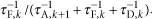Inserting these terms into equation (8), and using the boundary condition that at i=1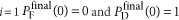(that is, for a nascent chain comprising one residue, the domain is denatured), this recursive relationship when solved equals equation (6).

### Numerical simulation of a collection of translating ribosomes

To simulate the stochastic nature of translation, it is necessary to consider the randomly distributed dwell times {τA} that the ribosome exhibits during its translation of an mRNA molecule. As the underlying Pi(τA) distribution of amino-acid addition times at codon i has not yet been experimentally determined, here we assume it to be exponentially distributed with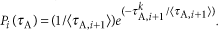For each ribosome, we constructed its {τA} by randomly sampling from this distribution, using an inverse transform sampling in which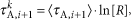where R is a random number selected from a uniform distribution in the range of (0,1). For each ribosome, this procedure results in 91 dwell times, representing a ribosome stochastically translating the protein G construct (Fig. 3a). This procedure was repeated 1,000 times, each yielding a unique {τA}, which represents the behaviour of 1,000 different synthesis events of this protein. Each {τA} was then inserted into equation (4) to yield their corresponding cotranslational folding curve (Fig. 6).

### Analysis of coarse-grained simulations

Mapping simulation timescales to experimental timescales. Low viscosity Langevin dynamics, as used in the coarse-grained simulations (Supplementary Methods), accelerate molecular dynamics while leaving the thermodynamic properties of the system unaltered. To map these accelerated kinetics to the experimentally relevant high-viscosity situation in aqueous media, we multiply the simulation time by the constant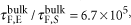the ratio of experimentally measured to calculated folding times.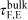is the experimentally (E) measured folding time of protein G in bulk solution and equals 2.4 ms (ref. 25), whereas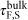is the average folding time from these simulations in the absence of the ribosome and equals 3.6 ns. This constant represents a linear scaling between the simulation time and the experimental time. Thus, in these simulations, when a new glycine residue is inserted into the growing chain during continuous translation every 90 ns (=6×106 integration time steps) of simulation time, this interval corresponds to an experimental time of τA=60 ms. Likewise, τF, τD and the other τA values reported in the main text are the results of multiplying their simulation times by this constant.

The probability of domain folding at equilibrium, PFE, was calculated from the Replica exchange simulations (Supplementary Methods). A given simulation conformation of protein G was considered to be folded, if its fraction of native contacts was greater than 50%, and otherwise was considered unfolded. The folded/unfolded time series for each replica was constructed using this definition, and the time series from replicas at different temperatures combined in the WHAM equations36 to calculate PFE. The stability of the folded state of protein G with respect to its denatured state, ΔGND, is equal to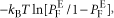where kB is Boltzmann's constant and T is the temperature.

The mean folding time τF of protein G equals the average of the set of first passage times {$τ F , i$} determined from temperature quench simulations at various nascent chain lengths (Supplementary Methods). τD is calculated as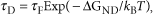where kB is Boltzmann's constant and T is the simulation temperature.

The probability of domain folding during continuous synthesis simulations, PF(i), was calculated as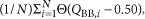where the summation is over the N-independent trajectories simulated for the given system, θ ($Q BB , i$−0.50) is the Heaviside step function that equals 1 if more than half of the native backbone contacts $Q BB , i$ in the structure of protein G are made in the last frame of the simulation at nascent chain length i and 0 otherwise.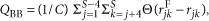where C is the number of native backbone contacts within the crystal structure, S (=56) is the number of interaction sites in protein G, and rjkF and rjk are, respectively, the spatial distances between interaction sites j and k in the crystal structure and the simulation structure. In this analysis, a native contact is identified in the crystal structure if any heavy atoms between residues j and k are within 4.5 Å of each other.

The standard error about the mean of τF was calculated by breaking the 152 independent folding trajectories into 15 sets of 10 or 11 τF,i values each, calculating the average value of each set and then calculating the standard deviation of the 15 averages divided by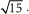To calculate the s.e.m. of ΔGND, the replica exchange simulation time-series data were broken into 5 independent sets, with approximately 20,000 points in each replica in each set. We then calculated ΔGND using each data set in the WHAM equations, and calculated the s.e.m., using these five ΔGND values. τD's s.e.m. was calculated using standard propagation of error equations.

How to cite this article: O'Brien, E. P. et al. Prediction of variable translation rate effects on cotranslational protein folding. Nat. Commun. 3:868 doi: 10.1038/ncomms1850 (2012).

## Change history

• ### 26 February 2013

A correction has been published and is appended to both the HTML and PDF versions of this paper. The error has not been fixed in the paper.

## References

1. 1

Hartl, F. U. & Hayer-Hartl, M. Converging concepts of protein folding in vitro and in vivo. Nat. Struct. Mol. Biol. 16, 574–581 (2009).

2. 2

Thirumalai, D., O'Brien, E. P., Morrison, G. & Hyeon, C. Theoretical perspectives on protein folding. Annu. Rev. Biophys. 39, 159–183 (2010).

3. 3

Nicola, A. V., Chen, W. & Helenius, A. Co-translational folding of an alphavirus capsid protein in the cytosol of living cells. Nat. Cell Biol. 1, 341–345 (1999).

4. 4

O'Brien, E. P., Christodoulou, J., Vendruscolo, M. & Dobson, C. M. New scenarios of protein folding can occur on the ribosome. J. Am. Chem. Soc. 133, 513–526 (2011).

5. 5

Elcock, A. H. Molecular simulations of cotranslational protein folding: fragment stabilities, folding cooperativity, and trapping in the ribosome. PLOS Comput. Biol. 2, 824–841 (2006).

6. 6

Fedyukina, D. V. & Cavagnero, S. Protein folding at the exit tunnel. Ann. Rev. Biophys. 40, 337–359 (2011).

7. 7

Ugrinov, K. G. & Clark, P. L. Cotranslational folding increases GFP folding yield. Biophys. J. 98, 1312–1320 (2010).

8. 8

Clark, P. L. & King, J. A newly synthesized, ribosome-bound polypeptide chain adopts conformations dissimilar from early in vitro refolding intermediates. J. Biol. Chem. 276, 25411–25420 (2001).

9. 9

Netzer, W. J. & Hartl, F. U. Recombination of protein domains facilitated by co-translational folding in eukaryotes. Nature 388, 343–9 (1997).

10. 10

Komar, A. A., Lesnik, T. & Reiss, C. Synonymous codon substitutions affect ribosome traffic and protein folding during in vitro translation. FEBS Lett. 462, 387–391 (1999).

11. 11

Tsai, C. J. et al. Synonymous mutations and ribosome stalling can lead to altered folding pathways and distinct minima. J. Mol. Biol. 383, 281–291 (2008).

12. 12

Kimchi-Sarfaty, C. et al. A 'silent' polymorphism in the MDR1 gene changes substrate specificity. Science 315, 525–528 (2007).

13. 13

Hopfield, J. J. Kinetic proofreading - new mechanism for reducing errors in biosynthetic processes requiring high specificity. Proc. Natl Acad. Sci. USA 71, 4135–4139 (1974).

14. 14

Ninio, J. Kinetic Amplification of enzyme discrimination. Biochimie 57, 587–595 (1975).

15. 15

Khushoo, A., Yang, Z., Johnson, A. E. & Skach, W. R. Ligand-driven vectorial folding of ribosome-bound human CFTR NBD1. Mol. Cell 41, 682–692 (2011).

16. 16

Uemura, S. et al. Real-time tRNA transit on single translating ribosomes at codon resolution. Nature 464, 1012–U73 (2010).

17. 17

Hsu, S. T. D. et al. Structure and dynamics of a ribosome-bound nascent chain by NMR spectroscopy. Proc. Natl Acad. Sci. USA 104, 16516–16521 (2007).

18. 18

Jackson, S. E. & Fersht, A. R. Folding of chymotrypsin inhibitor-2.1. Evidence for a 2-state transition. Biochemistry 30, 10428–10435 (1991).

19. 19

Zhang, G., Hubalewska, M. & Ignatova, Z. Transient ribosomal attenuation coordinates protein synthesis and co-translational folding. Nat. Struct. Mol. Biol. 16, 274–280 (2009).

20. 20

Fluitt, A., Pienaar, E. & Vijoen, H. Ribosome kinetics and aa-tRNA competition determine rate and fidelity of peptide synthesis. Comput. Biol. Chem. 31, 335–346 (2007).

21. 21

Qu, X. et al. The ribosome uses two active mechanisms to unwind messenger RNA during translation. Nature 475, 118–21 (2011).

22. 22

Schwartz, S. D. & Schramm, V. L. Enzymatic transition states and dynamic motion in barrier crossing. Nat. Chem. Biol. 5, 552–559 (2009).

23. 23

Dellago, C., Bolhuis, P. G., Csajka, F. S. & Chandler, D. Transition path sampling and the calculation of rate constants. J. Chem. Phys. 108, 1964–1977 (1998).

24. 24

Chung, H. S., McHale, K., Louis, J. M. & Eaton, W. A. Single-molecule fluorescence experiments determine protein folding transition path times. Science 335, 981–4 (2012).

25. 25

De Sancho, D., Doshi, U. & Munoz, V. Protein folding rates and stability: how much is there beyond size? J. Am. Chem. Soc. 131, 2074–2075 (2009).

26. 26

Kaiser, C. M., Goldman, D. H., Chodera, J. D., Tinoco, I. Jr & Bustamante, C. The ribosome modulates nascent protein folding. Science 334, 1723–7 (2011).

27. 27

Bingel-Erlenmeyer, R. et al. A peptide deformylase-ribosome complex reveals mechanism of nascent chain processing. Nature 452, 108–111 (2008).

28. 28

Gronenborn, A. M. et al. A novel, highly stable fold of the immunoglobulin binding domain of streptococcal protein-G. Science 253, 657–661 (1991).

29. 29

O'Brien, E. P., Hsu, S. T. D., Christodoulou, J., Vendruscolo, M. & Dobson, C. M. Transient tertiary structure formation within the ribosome exit port. J. Am. Chem. Soc. 132, 16928–16937 (2010).

30. 30

Kosolapov, A. & Deutsch, C. Tertiary interactions within the ribosomal exit tunnel. Nat. Struct. Mol. Biol. 16, 405–411 (2009).

31. 31

Purvis, I. J. et al. The efficiency of folding of some proteins is increased by controlled rates of translation invivo - a hypothesis. J. Mol. Biol. 193, 413–417 (1987).

32. 32

Young, R. & Bremer, H. Polypeptide-chain-elongation rate in escherichia-coli B-R as a function of growth-rate. Biochem. J. 160, 185–194 (1976).

33. 33

Agashe, V. R. et al. Function of trigger factor and DnaK in multidomain protein folding: Increase in yield at the expense of folding speed. Cell 117, 199–209 (2004).

34. 34

Czech, A., Fedyunin, I., Zhang, G. & Ignatova, Z. Silent mutations in sight: co-variations in tRNA abundance as a key to unravel consequences of silent mutations. Mol. Biosyst. 6, 1767–1772 (2010).

35. 35

Ninio, J. Alternative to the steady-state method: derivation of reaction rates from first-passage times and pathway probabilities. Proc. Natl Acad. Sci. USA 84, 663–7 (1987).

36. 36

Kumar, S., Bouzida, D., Swendsen, R. H., Kollman, P. A. & Rosenberg, J. M. The weighted histogram analysis method for free-energy calculations on biomolecules.1. the method. J. Comput. Chem. 13, 1011–1021 (1992).

## Acknowledgements

We thank Attila Szabo for stimulating discussions on chemical kinetic modelling and for proposing and deriving equation (6); Sophie Jackson for a careful reading of the manuscript; Robert Best for providing CHARMM source code for the double-well dihedral potential and Debye-Huckel electrostatic calculations; Changbong Hyeon for useful suggestions on modelling electrostatic interactions in coarse-grained models; and John Christodoulou for illuminating discussions about cotranslational folding. This work was supported by an NSF postdoctoral grant (EPO), BBSRC and the Wellcome Trust (MV and CMD), and the EPSRC (EPO, MV and CMD). This study utilized the high-performance computational capabilities of the Biowulf Linux cluster at the National Institutes of Health, Bethesda, Maryland. (http://biowulf.nih.gov).

## Author information

Authors

### Contributions

E.P.O., M.V., and C.M.D. designed the research. E.P.O. carried out the research and analysed the data. E.P.O., M.V., and C.M.D. interpreted the data and wrote the manuscript.

### Corresponding author

Correspondence to Christopher M. Dobson.

## Ethics declarations

### Competing interests

The authors declare no competing financial interests.

## Supplementary information

### Supplementary Information

Supplementary Tables S1-S2, Supplementary Methods and Supplementary References (PDF 579 kb)

## Rights and permissions

Reprints and Permissions

O'Brien, E., Vendruscolo, M. & Dobson, C. Prediction of variable translation rate effects on cotranslational protein folding. Nat Commun 3, 868 (2012). https://doi.org/10.1038/ncomms1850

• Accepted:

• Published:

• ### A code within the genetic code: codon usage regulates co-translational protein folding

• Yi Liu

Cell Communication and Signaling (2020)

• ### Genome-Scale Analysis of Perturbations in Translation Elongation Based on a Computational Model

• Doron Levin
•  & Tamir Tuller

Scientific Reports (2018)

• ### Cotranslational protein assembly imposes evolutionary constraints on homomeric proteins

• Eviatar Natan
• , Tamaki Endoh
• , Liora Haim-Vilmovsky
• , Tilman Flock
• , Guilhem Chalancon
• , Jonathan T. S. Hopper
• , Bálint Kintses
• , Peter Horvath
• , Lejla Daruka
• , Gergely Fekete
• , Csaba Pál
• , Balázs Papp
• , Erika Oszi
• , Zoltán Magyar
• , Joseph A. Marsh
• , Carol V. Robinson
• , Naoki Sugimoto
•  & Sarah A. Teichmann

Nature Structural & Molecular Biology (2018)

• ### Accurate prediction of cellular co-translational folding indicates proteins can switch from post- to co-translational folding

• Daniel A. Nissley
• , Ajeet K. Sharma
• , Nabeel Ahmed
• , Ulrike A. Friedrich
• , Günter Kramer
• , Bernd Bukau
•  & Edward P. O’Brien

Nature Communications (2016)

• ### Structural studies of the N-terminal fragments of the WW domain: Insights into co-translational folding of a beta-sheet protein

• Yuya Hanazono
• , Kazuki Takeda
•  & Kunio Miki

Scientific Reports (2016)